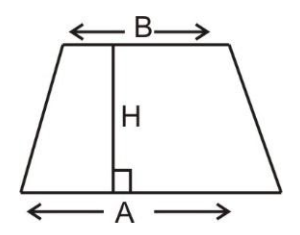QuestionAnswers

# A quadrilateral having one and only one pair of parallel sides is calledA) A parallelogramB) A kiteC) A rhombusD) TrapezoidsVerified
128.1k+ views
Hint: There we will be using the concept of quadrilateral, we can name any of the regular shapes by the number of sides or vertices or corners it has, a polygon which has 4 vertices or corners is called a quadrilateral.
Example: A chess board.

There are different types of quadrilaterals such as parallelogram, rectangle square, rhombus trapezoid and kite.
A) Parallelogram: It is quadrilateral with two pairs of parallel sides and opposite angles are equal.
B) Rectangle : It is quadrilateral with all the 4 angles of equal measure, each of them is .
C) Rhombus: It is a quadrilateral with all the four sides having equal lengths and the opposite sides are parallel.
D) Trapezoid: It is a quadrilateral with one and only one pair of parallel sides.Area of trapezoid = $\dfrac{{\left( {a + b} \right)}}{2} \times h$
a = long base
b = short base
h = altitude

Hence the correct option is option ‘D’.
Note: In these types of problems, properties of quadrilaterals must be known and types of quadrilateral will also help to recognize that which quadrilateral is that given one.
Some of the properties are as follows:
- Opposite angles are equal.
- Opposite sides are equal and parallel.
- Diagonals bisect each other.
- Sum of any two adjacent angles is $180^\circ$